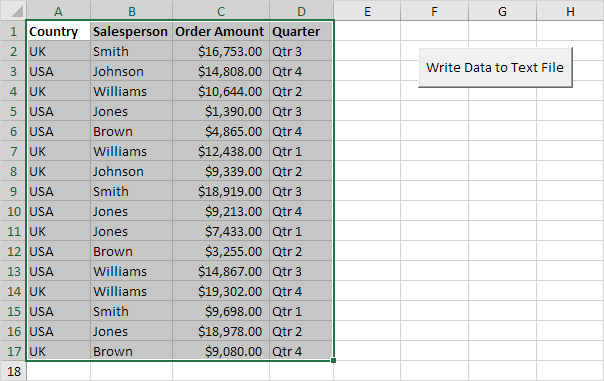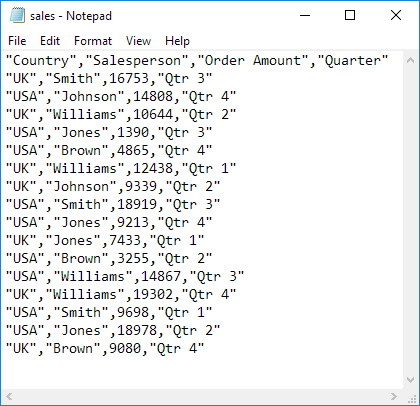# Write Data to Text File

Below we will look at a program in Excel VBA that writes an Excel range to a CSV (comma-separated-value) text file.

Situation:Place a command button on your worksheet and add the following code lines:

1. First, we declare a variable called myFile of type String, an object called rng of type Range, a variable called cellValue of type Variant, a variable called i of type Integer, and a variable called j of type Integer. We use a Variant variable here because a Variant variable can hold any type of value.

Dim myFile As String, rng As Range, cellValue As Variant, i As Integer, j As Integer

2. We need to specify the full path and the filename of the file.

myFile = Application.DefaultFilePath & "\sales.csv"

Note: the DefaultFilePath property returns the default file path. To change the default file location, on the File tab, click Options, Save.

3. We initialize the range object rng with the selected range.

Set rng = Selection

4. Add the following code line:

Open myFile For Output As #1

Note: this statement allows the file to be written to. We can refer to the file as #1 during the rest of our code. If the file already exists, it will be deleted and a new file with the same name will be created.

5. Start a Double Loop.

For i = 1 To rng.Rows.Count
For j = 1 To rng.Columns.Count

Note: rng.Rows.Count returns the number of rows (17 in this example) and rng.Columns.Count returns the number of columns (4 in this example).

6. Excel VBA writes the value of a cell to the variable cellValue.

cellValue = rng.Cells(i, j).Value

7. Add the following code lines to write the value of cellValue to the text file.

If j = rng.Columns.Count Then
Write #1, cellValue
Else
Write #1, cellValue,
End If

Explanation: due to the If Then Else statement, Excel VBA only starts a new line (Write #1, cellValue) when j equals the number of columns (last value in a row). To separate the values with a comma, use Write #1, cellValue, (with a comma).

8. Don't forget to close both loops.

Next j
Next i

9. Close the file.

Close #1

10. Select the data and click the command button on the sheet.

Result: